# HBSE 9th Class Maths Important Questions Chapter 3 Coordinate Geometry

Haryana State Board HBSE 9th Class Maths Important Questions Chapter 3 Coordinate Geometry Important Questions and Answers.

## Haryana Board 9th Class Maths Important Questions Chapter 3 Coordinate Geometry

Question 1.
Write the coordinates of each of the points A, B, C, D, E in figure.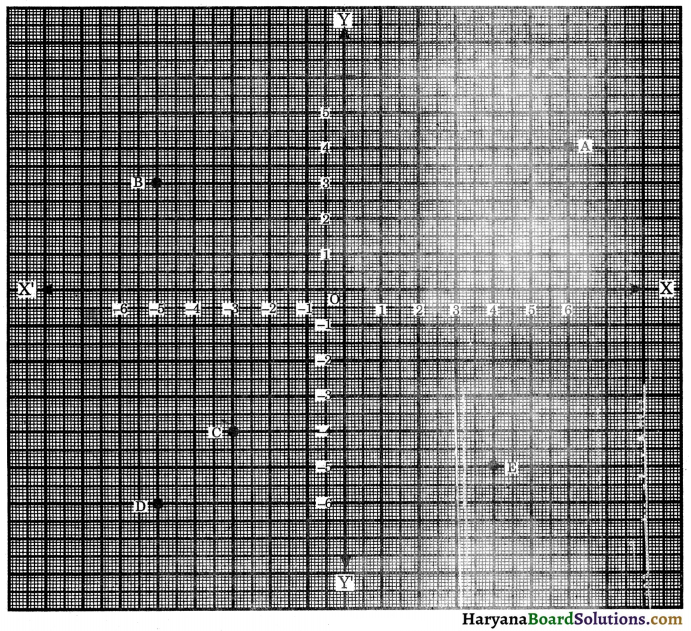Solution :
(i) Distance of the point A from the y-axis is 6 units and that of from x-axis is 4 units.
Hence, the coordinates of point A are (6, 4).
(ii) Distance of the point B from the y-axis is -5 units and that of from x-axis is 3 units.
Hence, the coordinates of point B are (- 5, 3).
(iii) Distance of the point C from the y-axis is – 3 units and that of from x-axis is 4 units.
Hence, the coordinates of point C are (- 3, – 4).
(iv) Distance of the point D from the y-axis is – 5 units and that of from x-axis is – 6 units.
Hence, the coordinates of point D are (- 5, – 6).
(v) Distance of the point E from the y-axis is 4 units and that of from x-axis is – 5 units.
Hence, the coordinates of point E are (4, – 5).Question 2.
Write down the abscissa, ordinate and coordinates of the point P as given in the figure.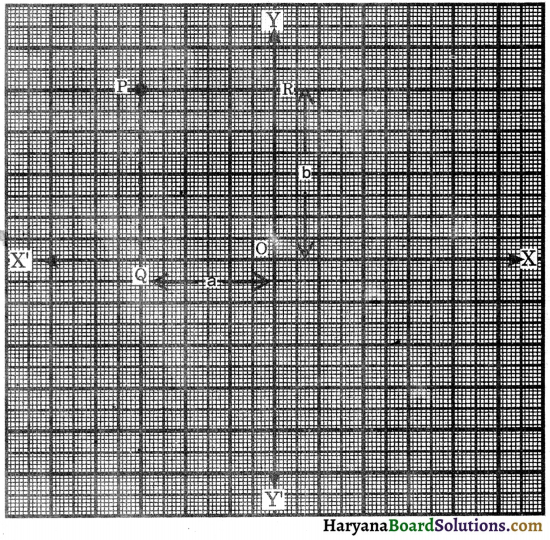Solution :
Abscissa of the point P = – PR = – OQ = – a
Ordinate of the point P = PQ = OR = b
Coordinates of the point P = (abscissa, ordinate) = (- a, b).

Question 3.
Plot the points (-2, 0), (2, 0), (2, 2), (0, 4) and (-2, 2) and join them in order. What figure do you get?
Solution :
Plotting the points A(-2, 0), B(2, 0), C(2, 2), D(0, 4) and E(-2, 2). Join A to B, B to C, C to D, D to E and E to A. The figure so formed is a pentagon as shown in the figure.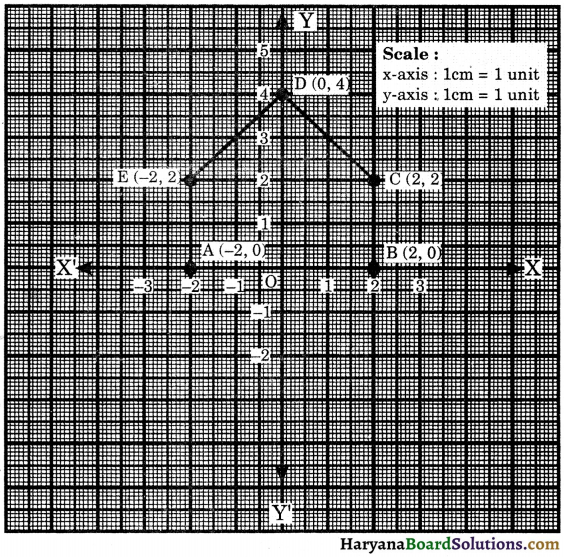Question 1.
Locate the points (3,0), (-3.5, 2.5), (5.5, – 3.5), (-3.5, – 4.5) and (0, – 5.5) in the Cartesian plane.
Solution :
We draw x-axis and y-axis on the graph paper. Using the scale 1 cm = 1 unit, the given points are plotted in the figure.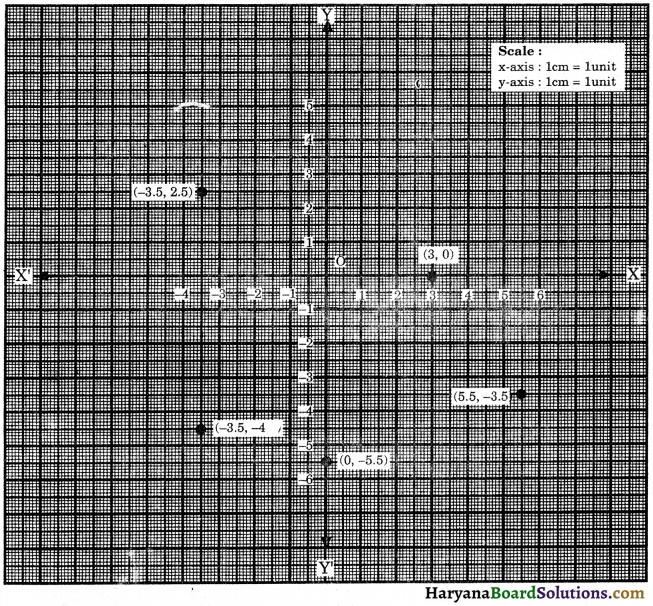Question 2.
Plot the points (x, y) given in the following table on the plane, choosing a suitable scale 1 cm = 0.5 unit on the axes.

 x 3.5 – 0.5 – 1.5 1.0 – 2.75 y 1.5 1.25 – 1.75 – 2.25 0.75

Solution :
The pairs of the numbers given in the table can be represented by the points (3.5, 1.5), (- 0.5, 1.25), (-1.5, – 1.75), (1.0, – 2.25), (- 2.75, 0.75). Scale is given that 1 cm = 0.5 unit. The locations of the points are shown by dots in the figure.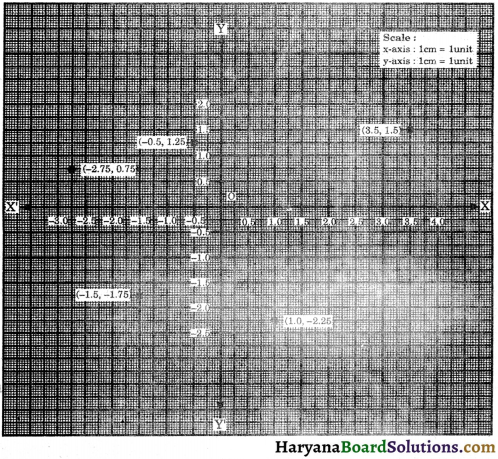Question 3.
Draw the triangle ABC, whose vertices are A(6, 2), B(3, – 1) and C(- 2, 4).
Solution :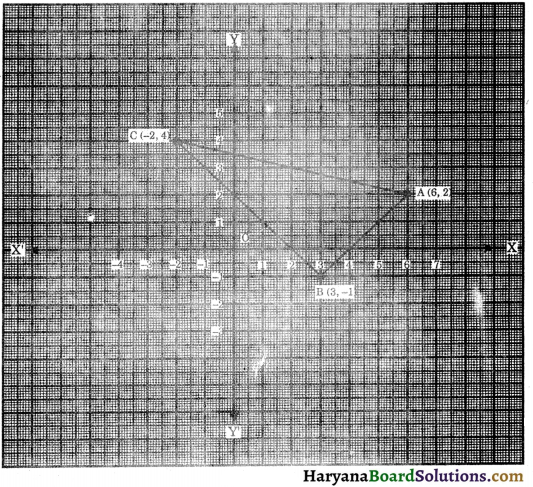With the axes, plot the points A(6, 2), B(3, – 1) and C(- 2, 4).
Joining the points A to B, B to C and C to A, then ΔABC is formed.Question 4.
Three vertices of a rectangle are (3, 2), (- 4, 2) and (-4, 5). Plot these points and find the coordinates of the fourth vertex. [NCERT Exemplar Problems]
Solution :
Plot the three vertices of the rectangle as A(3, 2), B(-4, 2), C(-4, 5).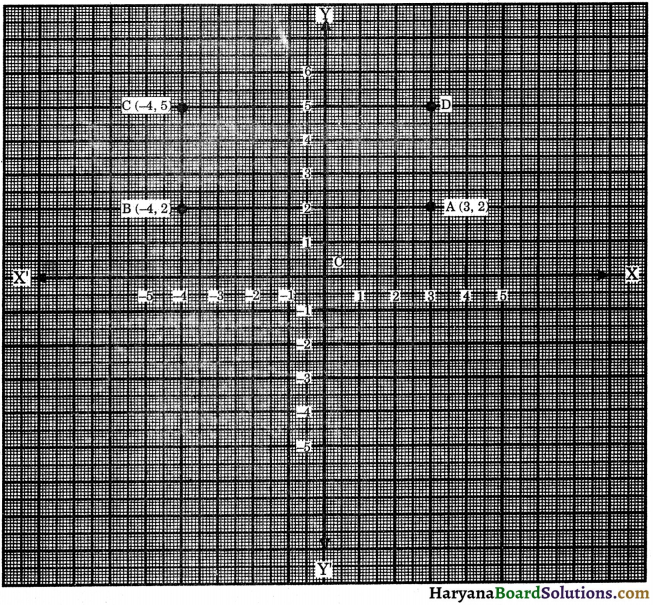Join A to B, B to Ć. Since sides of rectanges are equal and parallel, so through point C draw CD || AB and CD equal to AB and joing DA. Hence, coordinate of the point D are (3, 5).

Question 5.
The three vertices of a square ABCD taken in order are A(7, – 3), B(4, 1) and C(8, 4). Find the coordinates of the fourth vertex.
Solution :
Plotting the three vertices A(7, – 3), B(4, 1) and C(8, 4) of a square ABCD on the graph paper. Join A to B, B to C and complete the square as shown in the figure.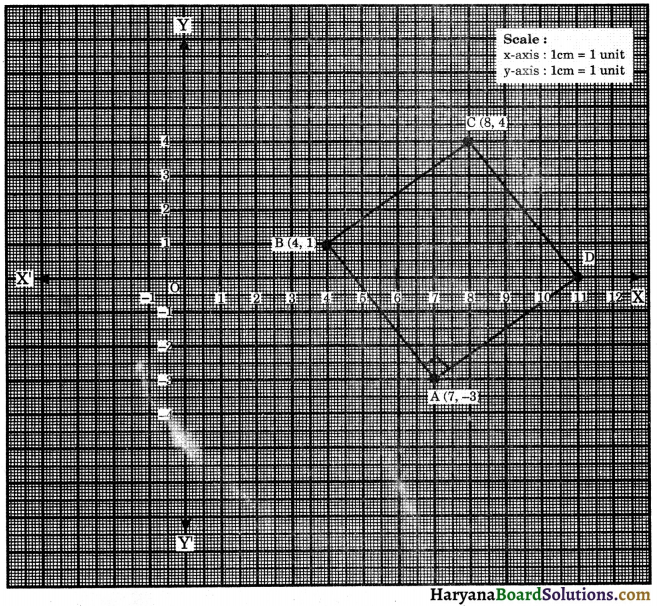So, fourth vertex Dis (11, 0).Question 6.
The length and breadth of a rectangle are a units and 6 units respectively, one vertex at origin. The length runs along positive x-axis and breadth on positive y-axis. Find the coordinates of the vertices of the rectangle.
Solution :
One vertex of a rectangle is origin. So its coordinates are A(0,0). Since, length runs along positive x-axis and breadth runs along positive y-axis. As length is a units and breadth is b units. So, the coordinates of IInd, IVth vertices are B(a, 0) and D(0, b) respectively. Distance of third vertex C from y-axis is a units and distance of third vertex C from x-axis is b units. So the coordinates of vertex Care (a, b). See in figure.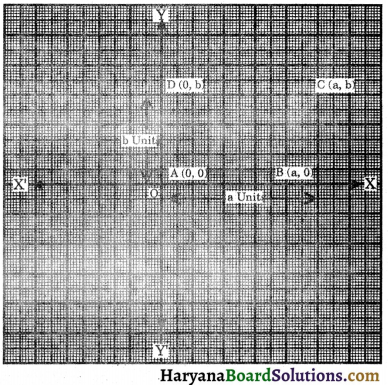Hence, the coordinates of the vertices of a rectangle are A(0,0), B(a,0), C(a,b) and D(0,b).

Question 1.
See the figure and write the following: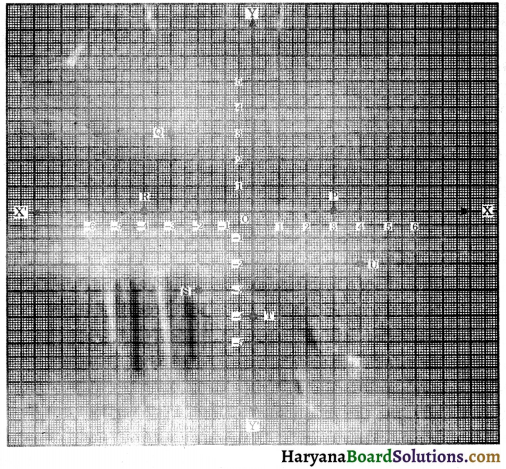(i) Coordinates of the point P.
(ii) Coordinates of the point R.
(iii) Abscissa of the point Q.
(iv) Ordinate of the point S.
(v) The point identified by the coordinates (0, – 4).
(vi) The point identified by the coordinates (4, – 2).
Solution:
(i) Distance of the point P from the y-axis is 3 units and that of from x-axis is 0 unit. Hence, the coordinates of point P are (3, 0).
(ii) Distance of the point R from the y-axis is – 4 units and that of from x-axis is 0 unit. Hence, the coordinates of point Rare (-4, 0).
(iii) Distance of the ponit Q from the y-axis is – 3 units. Hence, abscissa of the point Q is – 3
(iv) Distance of the point S from the x-axis is – 3 units. Hence, ordinate of the point S is – 3.
(v) The point identified by the coordinates (0, – 4) is ‘T’.
(vi) The point identified by the coordinates (4, – 2) is ‘U’.Question 2.
Write down the abscissa, ordinate and coordinates of the points A, B, C, D, E and F as given in the figure.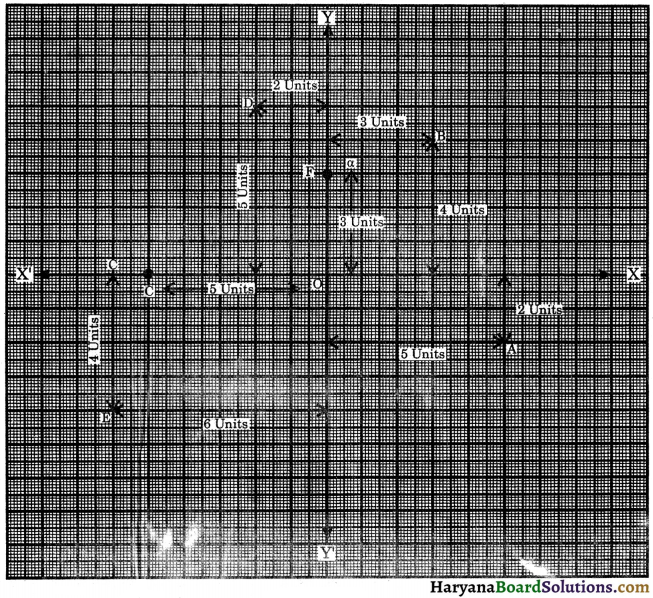Solution :
(i) Point A: Distance of the point A from the y-axis is 5 units. So, abscissa of the point A is 5. Distance of the point A from the x-axis is – 2 units. So, ordinate of the point A is – 2 units. Hence, coordinate of the point A are (5, – 2).
(ii) Point B: Abscissa of the point B is 3 units. Ordinate of the point B is 4 units. Hence, coordinates of the point B are (3, 4).
(iii) Point C: Abscissa of the point C is – 5 units. Ordinate of the point C is 0 unit. Hence, coordinates of the point C are (-5, 0).
(iv) Point D: Abscissa of the point D is – 2 units. Ordinate of the point D is 5 units. Hence, coordinates of the point D are (-2, 5).
(v) Point E: Abscissa of the point E is – 6 units. Ordinate of the point E is – 4 units. Hence, coordinates of the point E are (-6, – 4).
(vi) Point F: Abscissa of the point Fis 0. Ordinate of the point F is 3. Hence, coordinates of the point F are (0, 3).

Question 3.
Plot the following points and state whether they are collinear or not?
(i) A(-1, 2), B(2, 0) and C(5, – 2).
(ii) K(3, 2), L(- 2, – 2) and M(2, – 1).
(iii) O(0, 0), P(2, 2) and Q(5, 5).
[NCERT Exemplar Problems]
Solution :
(i) With the axes, plot the given points A(-1, 2), B(2, 0) and C(5, – 2).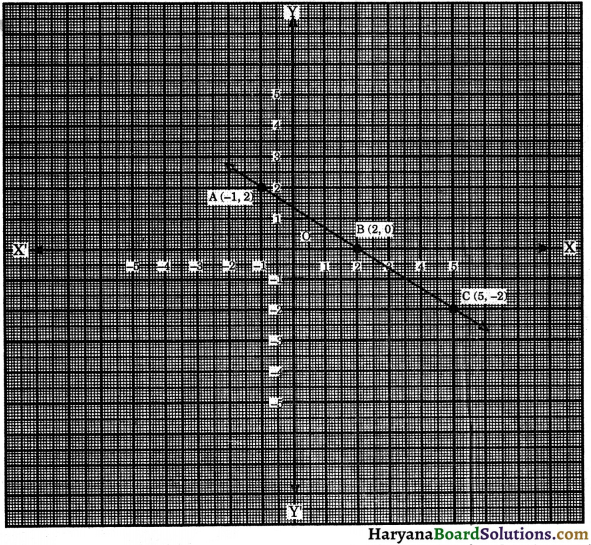Joining the points A to B and B to C, we get a straight line. Hence, the given points are collinear.

(ii) With the axes, plot the given points K(3, 2), L(-2, – 2) and M(2, – 1).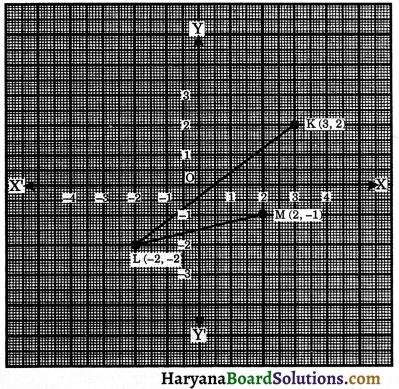Joining the points K to L and L to M, we do not get a straight line. Hence, the given points are not collinear.

(iii) With the axes, plot the given points 0(0,0), P(2, 2) and Q(5,5). Joining the points O to P and P to Q, we get a straight line. Hence, the given points are collinear.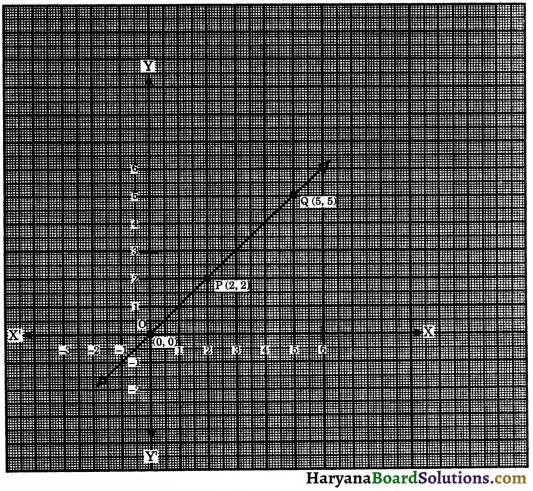Question 4.
Plot the following points and write the name of the quadrilateral so formed in each case :
(i) A(1, 1), B(6, 2), C(7, 6) and D(2, 5).
(ii) A(1, 1), B(2, 4), C(8, 4) and D(10, 1).
(iii) A(-2, – 2), B(-4, 2), C(-6, – 2) and D(-4, – 6).
Solution :
(i) With the axes, plot the given points A(1, 1), B(6, 2), C(7, 6) and D(2, 5).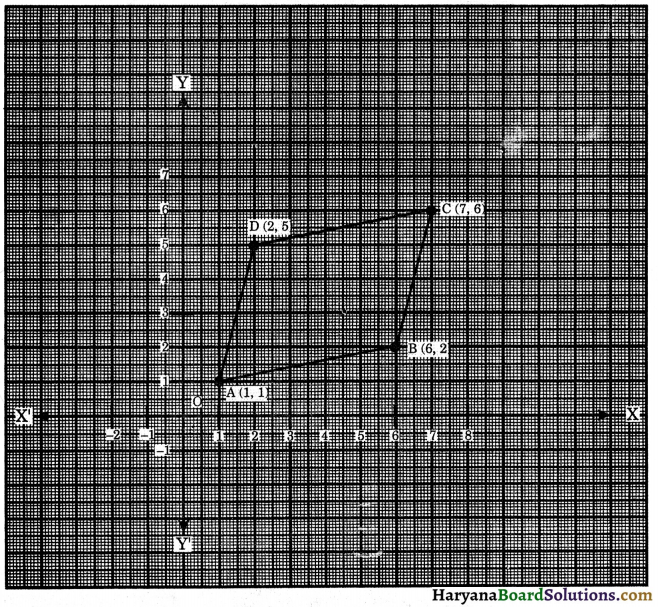Join A to B, B to C, C to D and D to A. The quadrilateral so formed is a parallelogram.

(ii) With the axes, plot the points A(1, 1), B(2,4), C(8,4) and D(10, 1).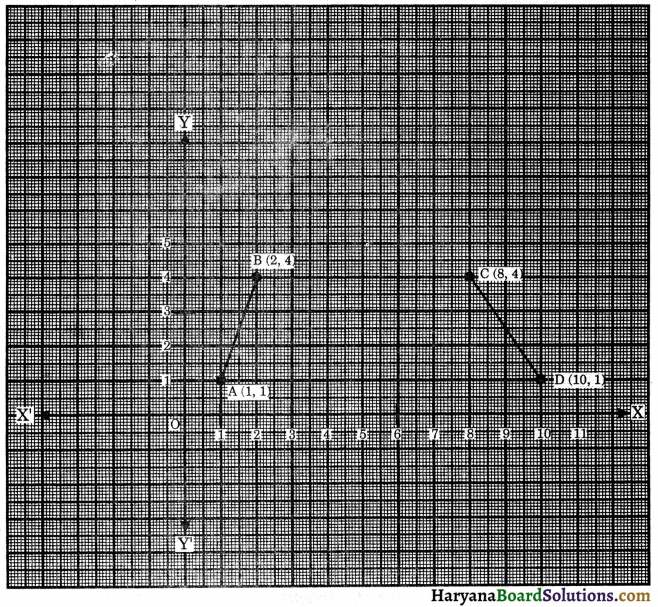Join A to B, B to C, C to D and D to A. The quadrilateral so formed is a trapezium.

(iii) With the axes, plot the given points A(-2, – 2), B(-4, 2), C(-6, – 2) and D(-4, – 6).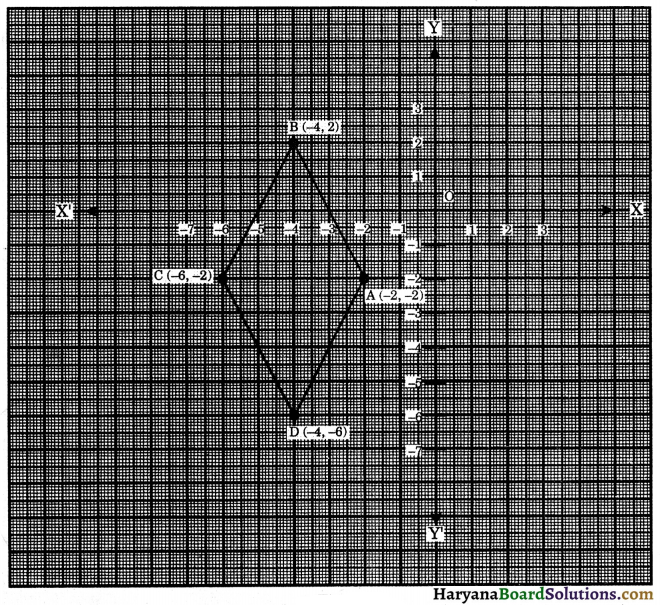Join A to B, B to C, C to D and D to A. The quadrilateral so formed is a rhombus.

Multiple Choice Questions

Choose the correct option in each of the following :

Question 1.
The point at which the x-axis and y-axis meet is called : [NCERT Exemplar Problems]
(a) the ordinate
(b) the abscissa
(c) the origin
Solution :
(c) the originQuestion 2.
The signs of abscissa and ordinate of a point in the quadrant IVth are :
(a) (+,+)
(b) (+, -)
(c) (-, +)
(d) (-, -)
Solution :
(b) (+, -)

Question 3.
The signs of abscissa and ordinate of a point in the quadrant II are:
[NCERT Exemplar Problems]
(a) (+, -)
(b) (-, -)
(c) (+, +)
(d) (-, +)
Solution :
(d) (-, +)

Question 4.
Ordinate of all points on the x-axis is :
[NCERT Exemplar Problems]
(a) – 1
(b) 1
(c) 0
(d) none of these
Solution :
(c) 0Question 5.
Abscissa of all points on the x-axis is :
[NCERT Exemplar Problems]
(a) 0
(b) – 1
(c) 1
(d) any number
Solution :
(d) any number

Question 6.
Abscissa of a point is negative in :
(d) IInd and IVth quarrants
Solution :

Question 7.
Ordinate of a point is positive in :
Solution :

Question 8.
The coordinates of the point lying on the negative side of y-axis at a distance of 7 units from the origin are :
(a) (0, 7)
(b) (0, – 7)
(c) (7, 0)
(d) (- 7, 0)
Solution :
(b) (0, – 7)Question 9.
The coordinates of the point lying on the negative side of x-axis at a distance of 3 units from the origin are :
(a) (0, 3)
(b) (0, – 3)
(c) (3, 0)
(d) (- 3, 0)
Solution :
(d) (- 3, 0)

Question 10.
The coordinates of the point lying on the positive side of y-axis at a distance of 5 units from the origin are :
(a) (5, 0)
(b) (- 5, 0)
(c) (0, 5)
(d) (0, – 5)
Solution :
(c) (0, 5)